Published: 30 June 2013

# An approach to health assessment for tools in milling machine

Bei Jikun1
Lu Chen2
Wang Zhipeng3
Wang Zili4
1, 2, 3, 4School of Reliability and Systems Engineering, Beihang University, Beijing, China
2State Key Laboratory of Virtual Reality Technology and Systems, Beijing, China
4, 2Science & Technology Laboratory on Reliability & Environmental Engineering, Beijing, China
Corresponding Author:
Lu Chen
Views 21

#### Abstract

Tool health is identified as the most significant index of the machining process, which directly influences the surface quality of work-piece. An online health monitoring for tools has become more crucial in manufacturing industries. In this study, a health assessment approach for tools in milling machine is presented. First, the vibration signal of tools is decomposed into a finite number of components called intrinsic mode function (IMF) by the empirical mode decomposition (EMD), which are regarded as the initial feature vector matrices. Second, Singular value decomposition (SVD) is used to extract the singular values of the matrices, which forms the feature vector for health assessment. Third, a Self-organizing mapping (SOM) network is introduced to map the extracted feature vectors into Minimum Quantization Error (MQE), and the Taguchi system is then employed to reduce the redundant features. Finally, the MQE is normalized into a confidence value (CV), representing the health status of the tools. A case study demonstrates that the proposed approach can effectively realize the health assessment for tools in milling machine by monitoring of the vibration signals.

## 1. Introduction

In recent years, monitoring of tool health, as one of the key techniques in modern manufacture, has attracted increased attention. During the manufacturing process, tool health status directly affects the surface finish and dimensional accuracy of the product, however, a health assessment based process can give a pre-warning, then repairs or replacements can be taken at the earliest or most convenient time with the minimum loss of productivity . Tool health assessment is therefore not only a diagnostics requirement to prevent machine-tool failure and production-material waste, but also plays crucial role which mainly affects the dimensional integrity, better performance and service-life of machined components .

Nowadays, many studies have been conducted on the tool wear monitoring, which mainly includes off-line and real-time measurement. The former usually performs the periodical measurements of tool state during machining process. Pham et al.  developed an electrical sensing circuit for wear measurement. Mizugaki  utilizes a laser range sensor, while Yan et al.  utilizes a machine vision system to measure the electrode wear. However, these approaches are extremely time-consuming since the machining process must be interrupted for periodical measurements. Therefore, the latter, a real-time monitoring approach, appears to be more pragmatic and has been well-developed in recent years. C. S. Ai et al.  proposed an approach to monitor milling tool wear using the cutting sound acoustic spectrum and the linear predictive cepstrum coefficient of the milling sound signal to monitor the milling tool wear. Martín P. Gómez et al.  investigated the correlation between the acoustic emission parameters and degrees of tool wear during the process and developed a strategy for detecting tool wear. G. Sutteret et al.  proposed a technique by analyzing the evolution of cutting force signal and the evolution of the friction coefficient. Mohammad et al.  proposed an approach to determine tool condition by using force and acoustic emission signal separately in micro-milling. Choi et al.  used acoustic emission and cutting forces in their attempt to develop a tool condition monitoring system for turning operations.

However, drawbacks of the present methods still exist. Noise signals or unknown influencing factors often disturb the acoustic emission, which makes it difficult to obtain the useful signals and characteristics. Force sensors are usually hard to assemble, and multiple force sensors are needed in some circumstances, resulting in cost-consuming impracticability for industrial applications . As for the other sensors with low costs, unfortunately, they often exhibit less sensitivity to cutting conditions and tool status. Compared with the above sensors, an accelerometer sensor is employed to acquire vibration signals in this study, which has the advantages on low cost and high sensitivity to tool wear.

The tool wear monitoring by vibration signal involves a significant issue: how to properly process and de-noise the monitored signals. Traditional signal processing techniques, including time-domain and frequency-domain analysis, are based on the assumption that the processing signals are stationary and linear. Unfortunately, vibration signals of worn tool are both nonlinear and non-stationary. In recent studies, several advanced time-frequency analysis techniques have been introduced to deal with non-stationary signals . Wigner-Ville distribution (WVD) is one of the most studied and applied methods in time–frequency analysis, which provides simple and clear energy spectrum diagram both in the time and frequency domains. However, cross term often shows in dealing with signals for its quadratic time-frequency nature. Wavelet transform, another time-frequency analysis techniques, has been extensive used in recent years. Signals are processed by a multi-scale analysis, which is suitable for non-stationary signals, but some deficiencies still exist. Firstly, energy leakage will occur when using wavelet transform to process signals due to the fact that wavelet transform is essentially an adjustable windowed Fourier transform . Secondly, once the wavelet function is selected, the result of wavelet transform would be the signal under a certain frequency band . Therefore, wavelet transform is not a self-adaptive signal processing method constitutionally.

The Empirical Mode Decomposition (EMD), proposed by N.E.Huang, provides an effective way to deal with nonlinear and non-stationary signals, it decomposes signals into intrinsic mode functions (IMFs) by their local time and scale characteristics, without a fixed pre-establish function, which makes it self-adaptive. Nowadays, EMD has been widely used in signal decomposition. Therefore, it is used for feature extraction in this paper.

Meanwhile, considering the periodic impulses usually exhibit in vibration signal while the tool wear occurs, singular value decomposition (SVD) of matrix can be used to extract features. According to the matrix theory, singular value is the nature characteristic of matrix and owns favorable stability. SVD has been broadly applied in regularization, noise reduction, signal estimation, signal detection. However, it still have some deficiencies, before reconstructing phase space, reconstruction parameters, such as lag time and embedding dimension must be determined, which will involve extensive computations. Thus, this construction method is unavailable in a real-time application . Here, the IMFs, resulted from EMD, are used to automatically form initial feature vector matrix, which is exactly the phase space. Therefore, an EMD based SVD technique is proposed as feature extraction method for tool vibration signal.

After feature extraction, a pattern recognition method is essential for assessment. Over the years, several techniques of pattern recognition have been well-developed. But, in general, the normal operation data sets are relatively easier to acquire, but it is hard to obtain whole-life data set representative when the tool runs for short time. Here, SOM based MQE chart, which quantifies the deviation degree of current process with only the normal process state space, is employed. SOM has proven to be powerful in analysis of complex industrial processes, which allows cluster structure of the data, monitoring of operation state, and novelty detection . The SOM has been successfully applied in various engineering applications . And for optimization, Taguchi method is introduced to choose the key features and discard the negative ones.

The main purpose of this study is to propose an approach to health assessment for milling tools based on the EMD, SVD, SOM-based MQE and Taguchi system. This paper is organized as follows: Section 2 introduces the methods of feature vector extraction using the EMD and SVD, MQE calculation using the SOM, feature vector optimization using the Taguchi method and the integrated process of tool health assessment. Section 3 describes the case studies to validate the proposed approach. Finally, Section 4 concludes this paper.

## 2. Methodology

### 2.1. Empirical mode decomposition

EMD is a self-adaptive method to decompose nonlinear and non-stationary signals. The advantage of EMD is that basis functions are derived from the analyzed signal itself. Hence, the signal analysis is adaptive in contrast to the traditional methods where basis functions are fixed. It decomposes the given signal into a series of IMFs through an iterative process called screening, each one, with distinct time scale .

First, Huang defined the IMF which should satisfy two conditions: (1) in the whole data set, the number of extrema and the number of zero crossings must either equal or differ at most by one; (2) at any point, the mean value of the upper envelope and lower envelope is zero . Then the sifting process is:

(a) For a given signal $s\left(t\right)$, construct its upper envelope and lower envelope by connecting all local maxima and local minima with cubic spline functions, respectively.

(b) Compute the envelopes mean $m\left(t\right)$ and extract the detail $h\left(t\right)=s\left(t\right)-m\left(t\right)$, regard $h\left(t\right)$ as new $s\left(t\right)$ and repeat the operation above until $h\left(t\right)$ satisfies the IMF conditions, then obtain the first IMF $im{f}_{1}\left(t\right)=h\left(t\right)$.

(c) Let the residual $r\left(t\right)=s\left(t\right)-h\left(t\right)$ be a new signal, repeat (a), (b) and (c), obtain the other orders IMFs.

EMD works like an adaptive high pass filter. It sifts out the fastest changing component of a composite signal first. The cut off frequency of the high pass filter is adaptive and data-driven. After the first filtering, the signal $s\left(t\right)$ can be expressed as:

1
$s\left(t\right)=im{f}_{1}\left(t\right)+{r}_{1}\left(t\right),$

where ${r}_{1}\left(t\right)$ is the low frequency component, compared with $im{f}_{1}\left(t\right).$ Repeating the decomposition on ${r}_{1}\left(t\right)$, then we obtain:

2
$s\left(t\right)=\sum _{i=1}^{n}im{f}_{i}\left(t\right)+{r}_{n}\left(t\right).$

Thus, each IMF contains lower-frequency oscillations than the prior-extracted one , $s\left(t\right)$ has been decomposed into $im{f}_{i}\left(t\right)$ and ${r}_{n}\left(t\right)$. Here, average trend of the signal is shown in the residual function ${r}_{n}\left(t\right);$$im{f}_{i}\left(t\right)$ contain different components of the signal from high frequencies to low ones, with the most important information of the given signal in the first several IMFs.

### 2.2. Singular value decomposition

SVD is an important algebra decomposition of matrix, which is widely used in signal processing and statistics . The SVD is defined as follows:

If $X$ is a real $m×n$ matrix, according to the SVD theorem, the matrix $X$ can be decomposed in the form:

3
$X=U\sigma {V}^{T},$

where $\sigma =\left[diag\left({\sigma }_{1},{\sigma }_{2},\cdots ,{\sigma }_{p}\right),O\right]$ or its transposition, which is decided by $m>n$ or $m; while $O$ is zero matrix; $p=min\left(m,n\right)$, and ${\sigma }_{1}\ge {\sigma }_{2}\ge \cdots \ge {\sigma }_{p}\ge 0$. These $\left(i=1,2,\dots ,p\right)$ are called the singular values of matrix $X$. $U\left(m×m\right)$ and $V\left(n×n\right)$ are orthogonal matrices and the columns of the orthogonal matrix $U$ and $V$ are called the left and right singular vectors. The singular values represent the importance of individual singular vectors in the composition of the matrix. In other words, a singular vector corresponding to the larger singular values have more information about the structure of the pattern embedded in the matrix than the other singular vectors.

Here, matrix of IMFs and the residual error is processed by singular value decomposition (SVD), singular values is obtain as $\left({E}_{1},{E}_{2},\cdots ,{E}_{n}\right)$, where $n$ is the number of IMFs and the residual error, normalized it as below:

4
${T}_{k}^{\text{'}}=\left({E}_{1}/\sum _{i=1}^{n}{E}_{i},{E}_{2}/\sum _{i=1}^{n}{E}_{i},\cdots ,{E}_{n}/\sum _{i=1}^{n}{E}_{i}\right).$

Considering the complexity of the signal RMS, kurtosis and peak value of the original signal are added as assist feature, in order to include both the entire signal and components features. RMS, kurtosis and peak value are figured out as $\left(R,K,P\right)$ and normalized as:

5
${T}_{k}^{\text{'}\text{'}}=\left(R/\mathrm{max}\left(R\right),K/\mathrm{max}\left(K\right),P/\mathrm{max}\left(P\right)\right).$

Finally, combine the above vector as:

6
${T}_{k}=\left({T}_{k}^{\text{'}},{T}_{k}^{\text{'}\text{'}}\right),$

where ${T}_{k}$ is the final normalized feature vector, which carries the information of tool wear. It can be used to classify different tool status, so as to assess the health.

### 2.3. MQE calculation based on SOM network

SOM is a kind of competed artificial neural network, which preserves mapping of the input data from its high-dimensional data space onto usually a two dimensional grid. Data points, which closely resemble each other, are located to nearby map neurons that form a two-dimensional lattice, each neuron is represented by a dimensional weight vector. Neurons of the map are connected to adjacent neurons by a neighborhood relation, which indicates the topology of the map .

During training procedure, take vector $X$ for example, the distance between it and all the weight vectors of SOM is computed by using distance measure as below. The neuron whose weight vector is closest to $X$ is called Best Matching Unit (BMU) :

7
$||X-{w}_{c}||=\underset{i}{\mathrm{m}\mathrm{i}\mathrm{n}}\left(||X-{w}_{i}||\right),$

where $c$ is the BMU, $||•||$ represent a distance measure, typically Euclidian distance. The weight vector of BMU is enhanced as well as its neighbors by the learning rule:

8
${w}_{i}\left(t+1\right)={w}_{i}\left(t\right)+\alpha \left(t\right)\cdot {h}_{BMU,i}\left(t\right)\left(x\left(t\right)-{w}_{i}\left(t\right)\right),$

where ${w}_{i}\left(t\right)$ represent the weight vector; $\alpha \left(t\right)$, the learning rate with the range of $0<\alpha \left(t\right)<1$; ${h}_{BMU,i}\left(t\right)$, neighborhood function determined by distance between $BMU$ and its neighbor.

After training, the neurons are grouped in clusters by their distance in the structure, which form a new kind of topology. When a test vector is inputted, the vector is compared with the weight vectors of all map units in SOM . Therefore, depending on how faraway the current data is deviating from the train data, a quantitative assessment index can be obtained by calculation of the Minimum Quantization Error (MQE) . $MQE$ value is figured out by:

9
$MQE=||{X}_{input}-{w}_{bmu}||,$

where ${X}_{input}$ is the input data vector, and ${w}_{bmu}$ is the weight vector of BMU. In this study, datasets of the tool that with mild wear are used as train data. Therefore, the $MQE$ shows how far the current tool status is deviating from the mild wear data. The health status can be quantified and visualized by following the trends of $MQE$.

### 2.4. Feature vector optimization using Taguchi method

Taguchi method is an important tool for robust design, which optimizes the product and process conditions that are minimally sensitive to the causes of variation. Meanwhile, the method can also be applied to the development of factorial experiments and the analysis of their associated outcomes.

To date, Taguchi method has become a well-recognized approach for analyzing the interaction effects while performing ranking and screening of various controllable factors. Moreover, this method is proven to be capable of solving a variety of problems involving continuous, discrete and qualitative design variables .

Here, Taguchi method is applied to optimize the feature vector. The optimization starts with the mild wear and severe wear tool feature vector, then the $MQE$ is figure out as preceding part of this text. After that, Taguchi method creates a standard orthogonal array (OA), which depends on the number of factors and levels needed. The optimum experimental conditions can be easily determined with the OA. Take an eight-factor-two-level OA for example (Table 1).

Table 1Taguchi orthogonal array

 Level Factor 1 2 3 4 5 6 7 8 Ex 1 1 1 1 1 1 1 1 1 2 1 1 1 1 1 2 2 2 3 1 1 2 2 2 1 1 1 4 1 2 1 2 2 1 2 2 5 1 2 2 1 2 2 1 2 6 1 2 2 2 1 2 2 1 7 2 1 2 2 1 1 2 2 8 2 1 2 1 2 2 2 1

In the OA, features have two levels. Level-1 represents the presence of a feature, while level-2 represents the absence of a feature. Here, nine MQEs are calculated with the combination of the features dictated by the OA and the larger-the-better signal-to-noise ratio (SNR) is calculated as follows:

10
${m}_{i}=-10\mathrm{log}\left[\frac{1}{n}\sum _{j=1}^{n}\frac{1}{MQ{E}_{j}}\right],$

where ${m}_{i}$ is the SNR for the $i$ th run of the OA, and $n$ is the sample size of each abnormality. Then, an average SNR at level-1 and level-2 of each feature is obtained and the gain is calculated as below :

11
$Gain=Mea{n}_{level1}-Mea{n}_{level2}.$

According to principle, features with positive gain are selected for the health assessment and the rest are discarded.

### 2.5. Health assessment using only normal condition datasets

As tools operate, the monitoring data obtained by accelerometer sensor is transferred to the trained SOM incessantly, which calculate the $MQE$ of the current status. In this approach, confidence value ($CV$), proposed by Qiu et al. , is introduced as an index to evaluate the health status. The $CV$ can be formulated as below:

12
$CV=\frac{c}{\sqrt{MQE}+c},$

where $c$ is scale parameter determined by the $MQE$ under the normal state and the predetermined $CV$, $MQE$ is just the $MQE$ under the monitoring data offered by SOM running.

According to the principles of SOM and $MQE$, mild wear signals with the similar features to the trained dataset, will gather around the normal cluster, which should have a minimum $MQE$ value relative to that of other faulty status. Along with the wear of tools, features of the signal turn to be more and more different from the trained data, therefore MQEs of them will become higher. $CV$ is inverse proportional to the $MQE$, so healthy tools get higher $CV$, and worn tools trend to be lower as well.

Therefore, the $CV$ of tools under normal conditions is expected to be close to 1, this value is defined as $C{V}_{pre}$. This initial value is mainly determined by experts in the field, and usually ranges from 0.99 to 0.90. $C{V}_{pre}$ can be denoted as:

13
$C{V}_{pre}=\frac{{c}_{0}}{\sqrt{mean\left(MQ{E}_{normal}\right)}+{c}_{0}},$

where $mean\left(MQ{E}_{normal}\right)$ is the average of all MQEs under normal conditions, and corresponds to the $mean\left(MQ{E}_{normal}\right)$, which is determined artificially. So, ${c}_{0}$ can be calculated as follows:

14
${c}_{0}=\frac{C{V}_{pre}\sqrt{mean\left(MQ{E}_{normal}\right)}}{1-C{V}_{pre}}.$

As $CV$ drops below the predetermined threshold, fault occurs. The CVs of the whole life of tool can be divided into three stages as Fig. 1.

Fig. 1Different stages of CVs during the whole life cycle of tool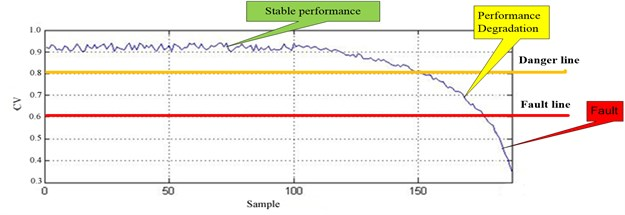### 2.6. Flow of the proposed method

The aforementioned methods consist of the health assessment process, as depicted in Fig. 2. It can be summarized as below.

Fig. 2Process of tool health assessment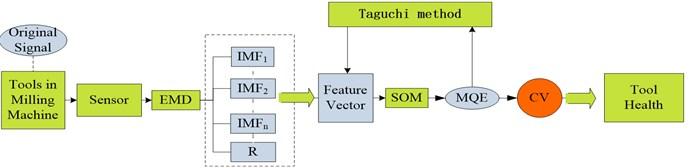The procedure can be divided into three parts:

Feature extraction: The vibration signal of a tool is decomposes into several IMFs and a residue ${\mathrm{r}}_{\mathrm{n}}$ using the EMD. The IMFs ${c}_{1},{c}_{2},\cdots ,{c}_{n}$ and ${r}_{n}$ are combined to construct an initial feature vector matrix. The SVD is then employed to obtain the singular values of the matrix, thereby forming the feature vector.

Pattern recognition: Prior to the real-time recognition, a Self-organizing mapping (SOM) network is utilized to map the extracted feature vectors into MQE. The Taguchi method is employed to generate the optimized feature vectors that are used to re-train the SOM, and the original MQEs are recalculated.

Health assessment: The obtained MQE is converted into a CV by a predetermined normalization function. Thus, the health state of the tool can be monitored by tracking its CVs curve.

## 3. Experimental verification

### 3.1. Experiment setup

The data in this study captures from runs on a milling machine. The cutting speed is set to 826 rev/min; two levels of cut depth are chosen as 0.75 mm and 1.5 mm; two levels of feed rate are taken as 0.25 mm/rev and 0.5 mm/rev which translate into 413 mm/min and 206.5 mm/min. Cast iron is used as the material. The size of the work-piece is 483 mm × 178 mm × 51 mm. In this research, two experiments with cut depth of 1.5 mm feed rate of 0.5 mm/rev, and cut depth of 0.75 mm feed rate of 0.25 mm/rev were chosen as example 1 and 2.

Fig. 3Sensor mounting position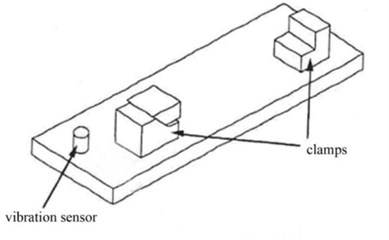A vibration sensor is mounted on the clamping device on the table, an accelerometer (model 7201-50, ENDEVCO) with a frequency range up to 13 KHz. Its signal is fed into an ENDEVCO 104 charge amplifier with sensitivity 5.71 and 100 mV/g output. The signal is then fed into an ITHACO 4302 Dual 24 dB octave filter with corner frequencies 400 Hz and 1 KHz. The signal is then fed through the PHOENIX CONTACT UMK-SE11,25 cable connector into the high speed data acquisition board of the computer.

Fig. 4Measure of flank wear VB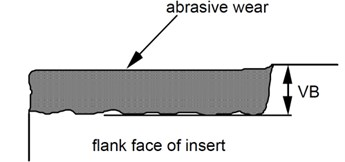The flank wear VB is measured as the distance from the cutting edge to the end of the abrasive wear on the flank face of the tool. The flank wear was observed during the experiments. The insert was taken out of the tool and the wear was measured with the help of a microscope.

### 3.2.1. Feature extraction

Tool original signal which is nonlinear and non-stationary is shown in Fig. 5. Here, EMD is employed to decompose it into IMFs.

Fig. 5A sample of EMD decomposition results of tool signal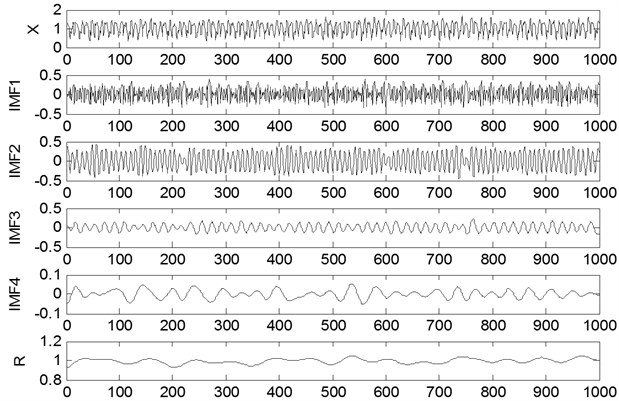Table 2Feature vector

 No. ${E}_{1}$ ${E}_{2}$ ${E}_{3}$ ${E}_{4}$ ${E}_{5}$ $R$ $K$ $P$ 1 0.4744 0.8642 0.1675 0.0049 0.0109 0.9012 0.7393 0.8068 2 0.4332 0.8964 0.0881 0.0329 0.0040 0.9491 0.7095 0.7624 3 0.4828 0.8629 0.1491 0.0102 0.0041 0.9568 0.7655 0.8175 4 0.5072 0.8504 0.1377 0.0233 0.0069 0.9341 0.7209 0.7720 5 0.4323 0.8862 0.1665 0.0064 0.0067 0.9151 0.7492 0.8014 6 0.4511 0.8832 0.1270 0.0161 0.0080 0.8899 0.7602 0.8130 7 0.5661 0.7986 0.2037 0.0135 0.0027 0.9064 0.7711 0.8280 8 0.4645 0.8684 0.1733 0.0093 0.0038 0.9386 0.7630 0.8181

According to the previous experiences, the first four IMFs which contain the most important information of the signal and a residual error are selected to form a feature matrix, SVD is employed to extract the feature. Then RMS, kurtosis and peak value are added to the feature vector. Table 1 shows 8 groups of normalized feature vectors from the tool with mild wear.

### 3.2.2. SOM-based MQE calculation with Taguchi method

After the extration, features are sent to SOM. SOM is trained by the vector of normal tools, with the trained SOM, MQE of each vector can be calculated. Here, Taguchi method is introduced in optimization, two tools with mild wear and wear of VB 0.76 mm, respectively, are send to Taguchi experiment. The result is shown as Table 3.

Table 3Result of Taguchi method

 Level Factor 1 2 3 4 5 6 7 8 ${m}_{i}$ Ex 1 1 1 1 1 1 1 1 1 0.11 2 1 1 1 1 1 2 2 2 –1.92 3 1 1 2 2 2 1 1 1 0.09 4 1 2 1 2 2 1 2 2 –0.65 5 1 2 2 1 2 2 1 2 –3.59 6 1 2 2 2 1 2 2 1 –3.67 7 2 1 2 2 1 1 2 2 –0.40 8 2 1 2 1 2 2 2 1 –2.82 9 2 1 1 2 2 2 1 2 –2.92 Mean level 1 –1.61 –1.31 –1.35 –2.05 –1.47 –0.21 –1.58 –1.57 Mean level 2 –2.05 –2.64 –2.08 –1.51 –1.98 –2.98 –1.89 –1.90 Gain 0.44 1.33 0.73 -0.54 0.51 2.77 0.31 0.33

According to the rules of Taguchi method, factor 4 with negative gain shoud be discarded. The rest are selected to constitute final vectors.

### 3.2.3. Health assessment

Here 110 samples from example 1 and 60 samples from example 2 are chosen to assess, every five samples are in the same VB. The CV and VB of each group are shown in Fig. 6 and 7.

Fig. 6CV and VB of the tools in example 1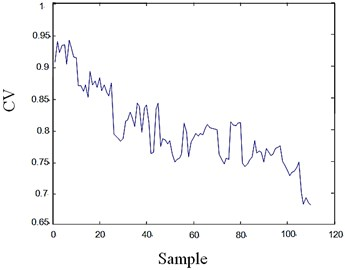a)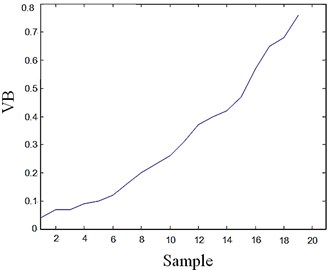b)

The blue curve in the left Figure shows trend of CV, the first ten samples with CVs above 9.0 indicate the tool is in good state, as the tool operating, CV falls at the same time, but it is still in the range of healthy state, as soon as the CV drop below a given threshold, quality of the work-piece will be influenced at high probability. VB is a index which directly represents the wear of tools. The trend of VB, expressed by the curve in the right Figure, clearly demonstrates that the tool health degenerates tracking as the CV.

Fig. 7CV and VB of the tools in example 2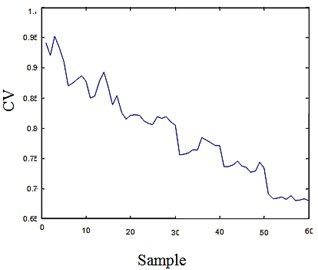a)b)

Example 2, as an assist group, shows the familiar trend with example 1, the VB curve proves the property of the result as well.

Thus it is clear that CV coincidently represents the health of tool and it is also distinctly proved that this approach to health assessment for tools is feasible.

## 4. Conclusions

This study presents a health assessment approach for tools in milling machine. Firstly, EMD is employed to decompose the signal of tool and feature vector is extracted with IMFs by SVD. Then SOM based MQE chart and Taguchi method are utilized to map the features into CV and optimize the feature. Its feasibility and efficiency has been verified in the experiment. Generally speaking, this approach focuses on the whole procedure which consists of signal processing, feature extraction, feature optimization and confidence value (CV) calculation. Vibration signal is used, which makes data acquisition easy, and the combination of EMD and SVD avoid some drawback in processing nonlinear and non-stationary signal. The employment of Taguchi method makes the result more accurate, and the optimization of the feature vector reduces computations as well, which is meaningful for real-time assessment.

Nevertheless, additional work is needed to further validate the method in wider applications. Meanwhile, relationship between CVs in different cutting condition ought to be investigated, and how to determine threshold, thereby accurately assessing health conditions, is also recognized as an issue.

11 February 2013
Accepted
03 June 2013
Published
30 June 2013
Keywords
health assessment
tool
empirical mode decomposition
singular value decomposition
self-organizing mapping network
Taguchi method
Acknowledgements

This research was supported by the National Natural Science Foundation of China (Grant Nos. 61074083, 50705005 and 51105019), as well as the Technology Foundation Program of National Defense (Grant No. Z132010B004).